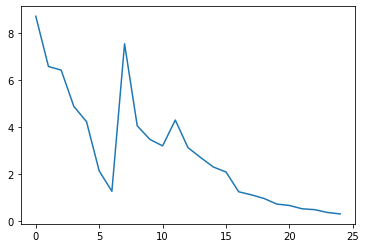This article was first published on T. Moudiki's Webpage - Python , and kindly contributed to python-bloggers. (You can report issue about the content on this page here)

Two weeks ago, I presented GPopt: a Python package for Bayesian optimization. In particular, I’ve presented a way to stop the optimizer and resume it later by adding more iterations.

This week, I present a way to save and resume, that makes the optimizer’s data persistent. Behind this saving feature, are hidden Python shelves which are – sort of – hash tables on disk.

We start by installing packages necessary for the demo.

```!pip install GPopt
!pip install matplotlib==3.1.3
```

Import packages.

```import GPopt as gp
import numpy as np
import matplotlib.pyplot as plt
```

Objective function to be minimized.

```# branin
def branin(x):
x1 = x
x2 = x
term1 = (x2 - (5.1*x1**2)/(4*np.pi**2) + (5*x1)/np.pi - 6)**2
term2 = 10*(1-1/(8*np.pi))*np.cos(x1)
return (term1 + term2 + 10)
```

Start the optimizer, and save on disk after 25 iterations.

```print("Saving after 25 iterations")
gp_opt3 = gp.GPOpt(objective_func=branin,
lower_bound = np.array([-5, 0]),
upper_bound = np.array([10, 15]),
n_init=10, n_iter=25,
save = "./save") # will create save.db in the current directory
gp_opt3.optimize(verbose=1)
print("current number of iterations:")
print(gp_opt3.n_iter)
gp_opt3.close_shelve()
print("\n")
print("current minimum:")
print(gp_opt3.x_min)
print(gp_opt3.y_min)
plt.plot(gp_opt3.max_ei)
```
```current number of iterations:
25

current minimum:
[3.17337036 2.07962036]
0.4318831996378023
```

#On this figure, we observe that there’s still room for advancement in the convergence of expected improvement (EI). We can add more iterations to the procedure by loading the saved object.

```print("---------- \n")
lower_bound = np.array([-5, 0]),
upper_bound = np.array([10, 15]))
print("current number of iterations:")
gp_optload.optimize(verbose=2, n_more_iter=190, abs_tol=1e-4) # early stopping based on expected improvement
print("current number of iterations:")
print("\n")
print("current minimum:")
```
```current number of iterations:
51

current minimum:
[9.44061279 2.48199463]
0.3991320518189241
```

#Now that the EI has effectively converged to 0, we can stop the optimization procedure.